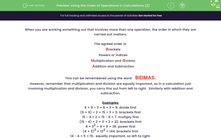# Using the Order of Operations in Calculations (2)

In this worksheet, students observe the order of priority in carrying out mixed operations.Key stage:  KS 3

Curriculum topic:   Number

Curriculum subtopic:   Use Conventional Notation for Priority of Operations

Difficulty level:#### Worksheet Overview

When you are working something out that involves more than one operation, the order in which they are carried out matters.

The agreed order is:

Brackets
Powers or indices
Multiplication and division

This can be remembered using the word    BIDMAS.

However, remember that multiplication and division are equally important, so in a calculation just involving multiplication and division, you carry this out from left to right.  Similarly with addition and subtraction.

Examples

6 + 9 ÷ 3 = 6 + 3 = 9. divide first
(6 + 9) ÷ 3 = 15 ÷ 3 = 5. brackets first
15 - 4 × 2 = 15 - 8 = 7. multiply first
(15 - 4) × 2 = 11 × 2 = 22. brackets first
4 × 32 = 4 × 9 = 36. power first
(4 × 3)2 = 122 = 144. brackets first

14 - 4 + 5 = 15.  equally important, so left to right.

### What is EdPlace?

We're your National Curriculum aligned online education content provider helping each child succeed in English, maths and science from year 1 to GCSE. With an EdPlace account you’ll be able to track and measure progress, helping each child achieve their best. We build confidence and attainment by personalising each child’s learning at a level that suits them.

Get started